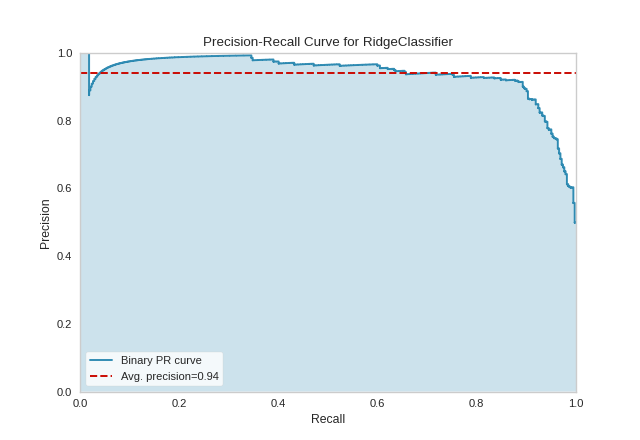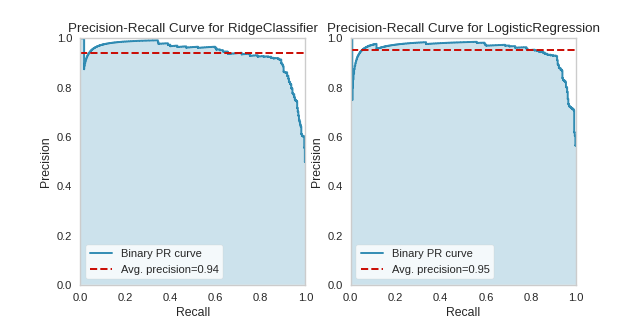# Precision-Recall Curves¶

Precision-Recall curves are a metric used to evaluate a classifier’s quality, particularly when classes are very imbalanced. The precision-recall curve shows the tradeoff between precision, a measure of result relevancy, and recall, a measure of how many relevant results are returned. A large area under the curve represents both high recall and precision, the best case scenario for a classifier, showing a model that returns accurate results for the majority of classes it selects.

## Binary Classification¶

from sklearn.linear_model import RidgeClassifier
from sklearn.model_selection import train_test_split as tts
from yellowbrick.classifier import PrecisionRecallCurve
from yellowbrick.datasets import load_spam

# Load the dataset and split into train/test splits
X, y = load_spam()

X_train, X_test, y_train, y_test = tts(X, y, test_size=0.2, shuffle=True)

# Create the visualizer, fit, score, and poof it
viz = PrecisionRecallCurve(RidgeClassifier())
viz.fit(X_train, y_train)
viz.score(X_test, y_test)
viz.poof()The base case for precision-recall curves is the binary classification case, and this case is also the most visually interpretable. In the figure above we can see the precision plotted on the y-axis against the recall on the x-axis. The larger the filled in area, the stronger the classifier is. The red line annotates the average precision, a summary of the entire plot computed as the weighted average of precision achieved at each threshold such that the weight is the difference in recall from the previous threshold.

## Multi-Label Classification¶

To support multi-label classification, the estimator is wrapped in a OneVsRestClassifier to produce binary comparisons for each class (e.g. the positive case is the class and the negative case is any other class). The Precision-Recall curve is then computed as the micro-average of the precision and recall for all classes:

from sklearn.ensemble import RandomForestClassifier
from sklearn.preprocessing import LabelEncoder, OrdinalEncoder
from sklearn.model_selection import train_test_split as tts
from yellowbrick.classifier import PrecisionRecallCurve
from yellowbrick.datasets import load_game

# Load dataset and encode categorical variables
X, y = load_game()
X = OrdinalEncoder().fit_transform(X)
y = LabelEncoder().fit_transform(y)

X_train, X_test, y_train, y_test = tts(X, y, test_size=0.2, shuffle=True)

# Create the visualizer, fit, score, and poof it
viz = PrecisionRecallCurve(RandomForestClassifier(n_estimators=10))
viz.fit(X_train, y_train)
viz.score(X_test, y_test)
viz.poof()A more complex Precision-Recall curve can be computed, however, displaying the each curve individually, along with F1-score ISO curves (e.g. that show the relationship between precision and recall for various F1 scores).

from sklearn.naive_bayes import MultinomialNB
from sklearn.preprocessing import LabelEncoder, OrdinalEncoder
from sklearn.model_selection import train_test_split as tts
from yellowbrick.classifier import PrecisionRecallCurve
from yellowbrick.datasets import load_game

# Load dataset and encode categorical variables
X, y = load_game()
X = OrdinalEncoder().fit_transform(X)
encoder = LabelEncoder()
y = encoder.fit_transform(y)

X_train, X_test, y_train, y_test = tts(X, y, test_size=0.2, shuffle=True)

# Create the visualizer, fit, score, and poof it
viz = PrecisionRecallCurve(
MultinomialNB(), per_class=True, iso_f1_curves=True,
fill_area=False, micro=False, classes=encoder.classes_
)
viz.fit(X_train, y_train)
viz.score(X_test, y_test)
viz.poof()## API Reference¶

Implements Precision-Recall curves for classification models.

class yellowbrick.classifier.prcurve.PrecisionRecallCurve(model, ax=None, classes=None, fill_area=True, ap_score=True, micro=True, iso_f1_curves=False, iso_f1_values=(0.2, 0.4, 0.6, 0.8), per_class=False, fill_opacity=0.2, line_opacity=0.8, **kwargs)[source]

Bases: yellowbrick.classifier.base.ClassificationScoreVisualizer

Precision-Recall curves are a metric used to evaluate a classifier’s quality, particularly when classes are very imbalanced. The precision-recall curve shows the tradeoff between precision, a measure of result relevancy, and recall, a measure of how many relevant results are returned. A large area under the curve represents both high recall and precision, the best case scenario for a classifier, showing a model that returns accurate results for the majority of classes it selects.

Parameters
modelthe Scikit-Learn estimator

An unfitted classification model to score the precision-recall curve on.

axmatplotlib Axes, default: None

The axes to plot the figure on. If None is passed in the current axes will be used (or generated if required).

classeslist

A list of class names for the legend. If classes is None and a y value is passed to fit then the classes are selected from the target vector. Note that the curves must be computed based on what is in the target vector passed to the score() method. Class names are used for labeling only and must be in the correct order to prevent confusion.

fill_areabool, default: True

Fill the area under the curve (or curves) with the curve color.

ap_scorebool, default: True

Annotate the graph with the average precision score, a summary of the plot that is computed as the weighted mean of precisions at each threshold, with the increase in recall from the previous threshold used as the weight.

microbool, default: True

If multi-class classification, draw the precision-recall curve for the micro-average of all classes. In the multi-class case, either micro or per-class must be set to True. Ignored in the binary case.

iso_f1_curvesbool, default: False

Draw ISO F1-Curves on the plot to show how close the precision-recall curves are to different F1 scores.

iso_f1_valuestuple , default: (0.2, 0.4, 0.6, 0.8)

Values of f1 score for which to draw ISO F1-Curves

per_classbool, default: False

If multi-class classification, draw the precision-recall curve for each class using a OneVsRestClassifier to compute the recall on a per-class basis. In the multi-class case, either micro or per-class must be set to True. Ignored in the binary case.

fill_opacityfloat, default: 0.2

Specify the alpha or opacity of the fill area (0 being transparent, and 1.0 being completly opaque).

line_opacityfloat, default: 0.8

Specify the alpha or opacity of the lines (0 being transparent, and 1.0 being completly opaque).

kwargsdict

Keyword arguments passed to the visualization base class.

Notes

Examples

>>> from yellowbrick.classifier import PrecisionRecallCurve
>>> from sklearn.model_selection import train_test_split
>>> from sklearn.svm import LinearSVC
>>> X_train, X_test, y_train, y_test = train_test_split(X, y)
>>> viz = PrecisionRecallCurve(LinearSVC())
>>> viz.fit(X_train, y_train)
>>> viz.score(X_test, y_test)
>>> viz.poof()

Attributes
target_type_str

Either "binary" or "multiclass" depending on the type of target fit to the visualizer. If "multiclass" then the estimator is wrapped in a OneVsRestClassifier classification strategy.

score_float or dict of floats

Average precision, a summary of the plot as a weighted mean of precision at each threshold, weighted by the increase in recall from the previous threshold. In the multiclass case, a mapping of class/metric to the average precision score.

precision_array or dict of array with shape=[n_thresholds + 1]

Precision values such that element i is the precision of predictions with score >= thresholds[i] and the last element is 1. In the multiclass case, a mapping of class/metric to precision array.

recall_array or dict of array with shape=[n_thresholds + 1]

Decreasing recall values such that element i is the recall of predictions with score >= thresholds[i] and the last element is 0. In the multiclass case, a mapping of class/metric to recall array.

draw(self)[source]

Draws the precision-recall curves computed in score on the axes.

finalize(self)[source]

Finalize the figure by adding titles, labels, and limits.

fit(self, X, y=None)[source]

Fit the classification model; if y is multi-class, then the estimator is adapted with a OneVsRestClassifier strategy, otherwise the estimator is fit directly.

score(self, X, y=None)[source]

Generates the Precision-Recall curve on the specified test data.

Returns
score_float

Average precision, a summary of the plot as a weighted mean of precision at each threshold, weighted by the increase in recall from the previous threshold.